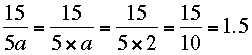SEARCH HOMEMath Central Quandaries & QueriesQuestion from Susan, a parent: Is "5a" considered an expression to be solved first, or do you simply go in PEMDAS order with the 15 divided by 5 in the following problem: Evaluate when a = 2 15 divided by 5aHi Susan,

You know that a = 2 so I would evaluate 5a first.PennyMath Central is supported by the University of Regina and The Pacific Institute for the Mathematical Sciences.